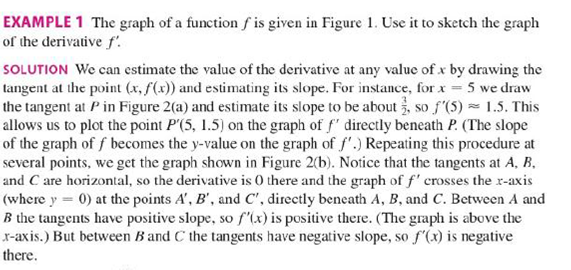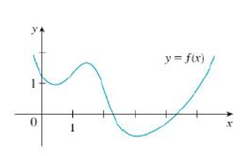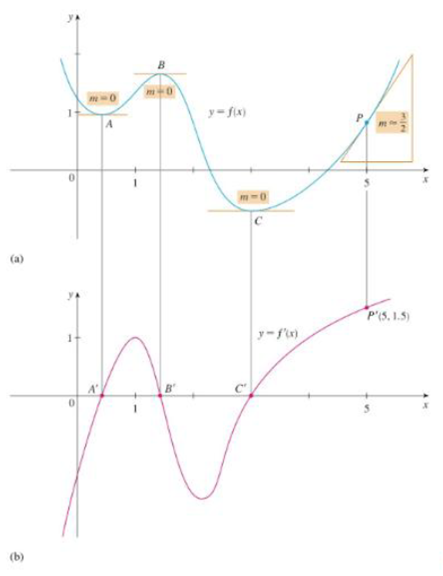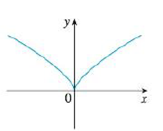Chapter 2.8, Problem 8E

Chapter
Section
Textbook Problem

Trace or copy the graph of the given function .f. (Assume that the axes have equal scales.) Then use the method of Example 1 to sketch the graph of f' below it.Example 1FIGURE 1FIGURE 2To determine

To sketch: The graph of f below the graph of f.

Explanation

From the point A to the left, the slope of the graph f is strictly negative which implies that the derivative graph f must have a functional value in negative.

From the given graph, it is observed that the graph has high sharpness at origin. So, there is no unique tangent line at origin. Thus, the given graph is not differentiable at (0,0). Therefore, the graph of f has a discontinuity at x=0

Still sussing out bartleby?

Check out a sample textbook solution.

See a sample solution

The Solution to Your Study Problems

Bartleby provides explanations to thousands of textbook problems written by our experts, many with advanced degrees!

Get Started

Proof Prove theorem 11.6.

Calculus (MindTap Course List)

In Exercises 4144, determine whether the statement is true or false. 43. 2356

Applied Calculus for the Managerial, Life, and Social Sciences: A Brief Approach

Simplify Simplify the rational expression. 22. y23y182y2+7y+3

Precalculus: Mathematics for Calculus (Standalone Book)

In Exercises 516, evaluate the given quantity. log5125

Finite Mathematics and Applied Calculus (MindTap Course List)